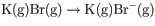# Problem: The following reaction can be written as the sum of two reactions, one of which relates to ionization energy and one of which relates to electron affinity:1. What is the reaction that corresponds to the first ionization energy of potassium, K?2. What is the reaction that corresponds to the electron affinity of bromine, Br?

###### FREE Expert Solution

We have to isolate the equations for ionization energy of potassium and electron affinity of bromine form this given equation.

K(g) + Br(g) → K+(g) + Br-(g)

Ionization energy is defined as the minimum energy required to remove a single valence electron from a gaseous atom.

Electron affinity is defined as the energy absorbed or released when a single electron is added to the valence shell of a gaseous atom.

79% (268 ratings)###### Problem Details

The following reaction can be written as the sum of two reactions, one of which relates to ionization energy and one of which relates to electron affinity:1. What is the reaction that corresponds to the first ionization energy of potassium, K?

2. What is the reaction that corresponds to the electron affinity of bromine, Br?